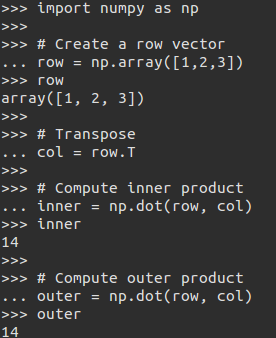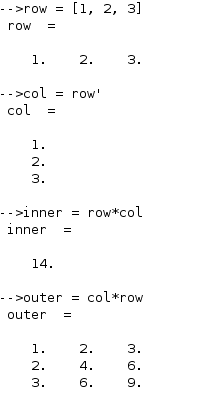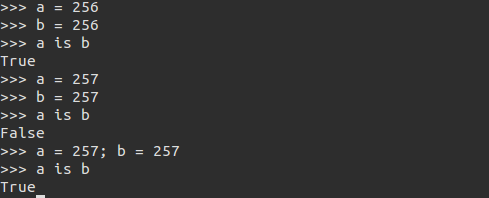## The great debate

There has been and would continue to be a healthy tiff between users of python and Scilab. While a lot of active development goes into the production of python as we see it now it is something on the lines of too many cooks make the broth difficult to eat . Although Scilab is still very nebular in the space of machine learning, I beleive that my project could be the ignition that it needed to conquer those territories.

SCILAB is an easy to use and productive computing environment for engineers and scientists and is dedicated to mathematical and technical computing.

In contrast, Python is a general-purpose programming language requiring add-on libraries for performing even basic mathematics.

Engineers and scientists need a programming language that expresses matrix and array mathematics directly instead of through generalized programming constructs. Matrix math in Python requires function calls, not natural operators, and you must keep track of the differences between scalars, 1-D arrays, and 2-D arrays. Even in the simplest Python code this can be difficult, as the hidden mistake in the following example shows. The same example written in the SCILAB language shows that SCILAB is the more natural way to express computational mathematics.As we can see in the code above row is a 1-d array. It looks like a row vector, but it doesn’t have enough dimensionality to say if it is a row or a column – it is just a 1-d array. Since there is no second dimension, the transpose in the third line has no effect. If we run the code similarly on Scilab, we see it is much more intuitive, and thus less prone to errors.Ultimately, linear algebra in SCIALB looks like linear algebra in a textbook. The same is true for data analytics, signal and image processing, control design, and other applications.

There are quartos and folios of python codes that are difficult to understand likeFor a complete list visit efforts by satwikkansal. In conclusion we can make out the following points :

• Scilab is math/computation and matrix-oriented language whereas python is a general-purpose language and numeric arrays and data types not part of the core language.

• Beginners write math as they would on paper for Scilab whereas python users must become programmers before they can be productive.

Saying that python is never usable for data science would be a very unfair statement since the amount of code invested into easing the process out is immense. Though it depends on each user, Scilab is much well suited for a person not looking at the exact construct of a program but the domain.

### Authors:

• Soumitra Agarwal